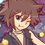# Number Theory (Thailand Math POSN 2nd round)

Write a full solution

Theorems allowed to use: - Divisibility, gcd, lcm, Prime numbers - Modular arithmetic - Chinese Remainder Theorem - Complete Residue System modulo n - Reduced Residue System modulo n - Euler's Theorem - Fermat's Little Theorem - Wilson's Theorem

1.) Prove that there are no positive integers $n$ such that

$2558^{n} \equiv 1 \pmod{2000^{n} - 1}$

2.) Find the largest positive integer $n$ such that for all positive integers$a$,

$a^{9} \equiv a \pmod{n}$

3.) Find all solutions to linear congruence

$2557x \equiv 2015 \pmod{1200}$

4.) Let $p$ be a prime number and $N = \displaystyle \frac{10^{p} - 1}{9}$, prove that

$N\times 10^{8p} + 2N\times 10^{7p} + 3N \times 10^{6p} + \dots + 9N \equiv 123456789 \pmod{p}$

5.) Let $p$ be a prime number, prove that the congruence

$x^{2} \equiv -1 \pmod{p}$

has a solutions if and only if $p = 2$ or $p \equiv 1 \pmod{4}$.

This note is a part of Thailand Math POSN 2nd round 2015Note by Samuraiwarm Tsunayoshi
6 years ago

This discussion board is a place to discuss our Daily Challenges and the math and science related to those challenges. Explanations are more than just a solution — they should explain the steps and thinking strategies that you used to obtain the solution. Comments should further the discussion of math and science.

When posting on Brilliant:

• Use the emojis to react to an explanation, whether you're congratulating a job well done , or just really confused .
• Ask specific questions about the challenge or the steps in somebody's explanation. Well-posed questions can add a lot to the discussion, but posting "I don't understand!" doesn't help anyone.
• Try to contribute something new to the discussion, whether it is an extension, generalization or other idea related to the challenge.
• Stay on topic — we're all here to learn more about math and science, not to hear about your favorite get-rich-quick scheme or current world events.

MarkdownAppears as
*italics* or _italics_ italics
**bold** or __bold__ bold
- bulleted- list
• bulleted
• list
1. numbered2. list
1. numbered
2. list
Note: you must add a full line of space before and after lists for them to show up correctly
paragraph 1paragraph 2

paragraph 1

paragraph 2

[example link](https://brilliant.org)example link
> This is a quote
This is a quote
    # I indented these lines
# 4 spaces, and now they show
# up as a code block.

print "hello world"
# I indented these lines
# 4 spaces, and now they show
# up as a code block.

print "hello world"
MathAppears as
Remember to wrap math in $$ ... $$ or $ ... $ to ensure proper formatting.
2 \times 3 $2 \times 3$
2^{34} $2^{34}$
a_{i-1} $a_{i-1}$
\frac{2}{3} $\frac{2}{3}$
\sqrt{2} $\sqrt{2}$
\sum_{i=1}^3 $\sum_{i=1}^3$
\sin \theta $\sin \theta$
\boxed{123} $\boxed{123}$

## Comments

Sort by:

Top Newest

Solution to Q-4:

From Fermat's Little Theorem, we know that, for any integer $a$ and a prime $p$, we have $a^p\equiv a\pmod{p}$. Then,

$10^p\equiv 10\pmod{p}\implies 10^p-1\equiv 9\pmod{p}\implies N=\frac{10^p-1}{9}\equiv 1\pmod{p}~,p\neq 3$

We have $p\neq 3$ in that case since $\gcd(9,3)=3\neq 1$ and $3$ is the only prime that is not coprime to $9$.

For $p=3$, using divisibility rule of $3$, we have $N=\dfrac{10^3-1}{9}=111\equiv 0\pmod{3}$. Hence, we combine these two results as (with prime $p$),

$N=\frac{10^p-1}{9}\equiv \begin{cases}1\pmod{p}~,~p\neq 3\\ 0\pmod{p}~,~p=3\end{cases}~\ldots~(i)$

Trivial Observation 1: We note beforehand that $123456789\equiv 0\pmod{3}$ using the divisibility rule of $3$. As such, if we have $x\equiv 0\pmod{3}$, we can also write that as $x\equiv 123456789\pmod{3}$ for any integer $x$.

Now, for positive integral $n$, $10^n\in\mathbb{Z}$ and hence, by FLT, we have $10^{np}\equiv 10^n\pmod{p}$ for all primes $p$ and positive integral $n$. We use this result to form a modular congruence equation with the LHS of the statement to be proved modulo prime $p$ as,

$\textrm{LHS}\equiv N(10^8+2\times 10^7+3\times 10^6+\ldots +9)\equiv 123456789N\pmod{p}$

Using $(i)$, this becomes,

$\textrm{LHS}\equiv\begin{cases} 123456789\pmod{p}~,~p\neq 3\\ 0\pmod{p}~,~p=3\end{cases}$

Using Trivial Observation 1, this becomes,

$\textrm{LHS}\equiv 123456789\pmod{p}=\textrm{RHS}$

$\therefore\quad N\times 10^{8p} + 2N\times 10^{7p} + 3N \times 10^{6p} + \dots + 9N \equiv 123456789 \pmod{p}$

- 6 years ago

Log in to reply

prob 1 : first assume there is a positive number n such that 2558^n =1 (mod 2000^n -1) or, 2558^n-1=0( mod 2000^n-1) that means 2000^n-1 divides 2558^n-1 now , let p be the maximum prime divisor of 2000^n -1 so. 2000^n=1(mod p) let this p be also a maximum prime divisor of 2558^n-1 so. 2558^n=1 (mod p) then , 2000^n =2558^n or 2000= 2558 and it is not possible . So there are no integer n such that 2558^n=1(mod 2000^n-1)

- 5 years, 6 months ago

Log in to reply

In your solution, it seems to me that you assume that $a^n\equiv b^n\pmod{p}\implies a=b$ where $a,b,n$ are positive integers and $p$ is a prime. But that doesn't necessarily hold true.

Consider $a=3,b=4,p=11,n=10$. We have $a\neq b$ but $a^n\equiv b^n\pmod{p}$.

- 5 years, 6 months ago

Log in to reply

Solution to Q no 3 : 2557x=2015(mod 1200) Since gcd (2557,1200)=1 there is an unique solution (mod 1200) By using the Euclidean Algorithm to write 1 as the linear combination of 2557 and 1200, such as 1200×228-2557(-107)=1 here 2557(-107)=1(mod 1200) so, 2557(-107×2015)=2015 (mod 1200) Since , -107×2015 = 395 (mod 1200) We conclude that x=395 ( modulo 1200) is an unique solution . so x =395

- 5 years, 6 months ago

Log in to reply

Nice solution. Also this question can be done by the CRT

- 5 years, 3 months ago

Log in to reply

×

Problem Loading...

Note Loading...

Set Loading...